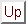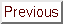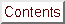Next: Note about the validity Up: The CONDOR unconstrained algorithm. Previous: The CONDOR unconstrained algorithm.   Contents

# The bound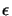.

See Section 3.4.2 to know about.
If we have updated the value of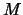less than 10 times, we will set(see Section 3.4.1 to know about). This is because we are not sure of the value ofif it has been updated less than 10 times.
If the step size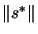we have computed at step 3 of the CONDOR algorithm is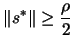, then.
Otherwise,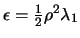, where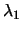is an estimate of the slope ofaround(see Section 4.10 to know how to compute).
We see that, when the slope is high, we permit a more approximative (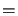big value of) model of the function.

Frank Vanden Berghen 2004-04-19